# Cartan theorem

(diff) ← Older revision | Latest revision (diff) | Newer revision → (diff)

Cartan's theorem on the highest weight vector. Letbe a complex semi-simple Lie algebra, let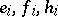,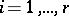, be canonical generators of it, that is, linearly-independent generators for which the following relations hold: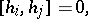where,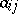are non-positive integers when,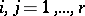,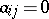implies, and letbe the Cartan subalgebra ofwhich is the linear span of. Also letbe a linear representation ofin a complex finite-dimensional space. Then there exists a non-zero vectorfor which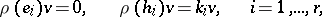where the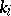are certain numbers. This theorem was established by E. Cartan . The vectoris called the highest weight vector of the representationand the linear function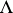ondefined by the condition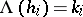,, is called the highest weight of the representationcorresponding to. The ordered setis called the set of numerical marks of the highest weight. Cartan's theorem gives a complete classification of irreducible finite-dimensional linear representations of a complex semi-simple finite-dimensional Lie algebra. It asserts that each finite-dimensional complex irreducible representation ofhas a unique highest weight vector (up to proportionality), and that the numerical marks of the corresponding highest weight are non-negative integers. Two finite-dimensional irreducible representations are equivalent if and only if the corresponding highest weights are the same. Any set of non-negative integers is the set of numerical marks of the highest weight of some finite-dimensional complex irreducible representation.

## Contents

How to Cite This Entry:
Cartan theorem. Encyclopedia of Mathematics. URL: http://encyclopediaofmath.org/index.php?title=Cartan_theorem&oldid=15979
This article was adapted from an original article by V.L. Popov (originator), which appeared in Encyclopedia of Mathematics - ISBN 1402006098. See original article